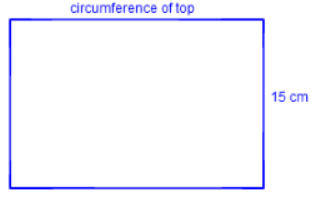### Home > GC > Chapter 11 > Lesson 11.1.1 > Problem11-9

11-9.

The lateral surface of a cylinder is the surface connecting the bases. For example, the label from a soup can could represent the lateral surface of a cylindrical can. If the radius of a cylinder is $4 \text{ cm}$ and the height is $15 \text{ cm}$, find the lateral surface area of the cylinder. Note: It may help you to think of “unrolling” a soup can label and finding the area of the label.$\text{circumference of top} = 2πr = 8πcm$
$\left(8π\right)\left(15\right) = 120π \ 377 \text{ cm}^{2}$